# 数字图像处理—表达与描述

## （一）背景知识

1. 图像分割结果是得到了区域内的像素集合，或位于区域边界上的像素集合，这两个集合是互补的。

2. 与分割类似，图像中的区域可用其内部(如组成区域 的像素集合)表示，也可用其外部（如组成区域边界的 像素集合）表示。

3. 一般来说，如果关心的是区域的反射性质，如灰 度、颜色、纹理等，常用内部表示法；如果关心的是区域形状，则选用外部表示法。

4. 表示是直接具体地表示目标，好的表示方法应具有节省存储空间、易于特征计算等优点。

5. 描述是较抽象地表示目标。好的描述应在尽可能区 别不同目标的基础上对目标的尺度、平移、旋转等不敏感，这样的描述比较通用

6. 描述可分为对边界的描述和对区域的描述。此外， 边界和边界或区域和区域之间的关系也常需要进行描 述

7. 表示和描述是密切联系的。表示的方法对描述很重要，因为它限定了描述的精确性；而通过对目标的描 述，各种表示方法才有实际意义

8. 表示和描述又有区别，表示侧重于数据结构，而描述侧重于区域特性以及不同区域间的联系和差别。

9. 对目标特征的测量是要利用分割结果进一步从图像中获取有用信息，为达到这个目的需要解决两个关键问题：
a. 选用什么特征来描述目标
b. 如何精确地测量这些特征

10. 常见的目标特征分为灰度（颜色）、纹理和几何形状特征等。其中，灰度和纹理属于内部特征，几何形状属于外部特征。

## （二）表示

#### 2.1 链码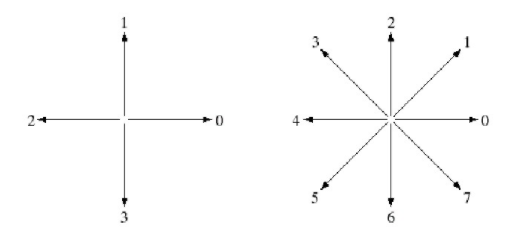1. 给每一个线段边界一个方向编码。
2. 有4链码和8链码两种编码方法。
3. 从起点开始，沿边界编码，至起点被重新碰到，结束一个对象的编码。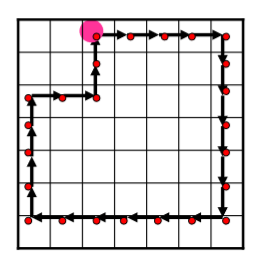4-链码：000033333322222211110011

1）链码相当长
2）噪音会产生不必要的链码

1）加大网格空间
2）依据原始边界与结果的接近程度， 来确定新点的位置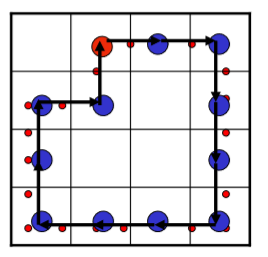4-链码：003332221101

1）由于起点的不同，造成编码的不同
2）由于角度的不同，造成编码的不同

1）从固定位置作为起点(左上)开 始编码
2）通过使用链码的差分代替码字本身的方式

循环差分为：33133030
循环差分：1 -2 = -1(3)      3 -0 = 3
0 -1 = -1(3)     3 -3 = 0
1 -0 = 1           2 -3 = -1(3)
0 -1 = -1(3)     2 -2 = 0

c = fchcode(b, conn, dir)

1. 参数coon指明链码的连接方式：值可为 4 或 8(默认的)。当边界不包含对角转换时，值设 conn 为 4 是有效的。
2. 参数 dir 指明输出链码的方向。如果指定为 ‘same’，链码方向与 b中点的方向相同。用’reverse’将导致链码方向相反。默认为’same’。

f = imread('D:\数字图像处理\第十一章学习\Fig1103(a).tif');
h = fspecial('average', 9);
g = imfilter(f, h, 'replicate');
gB = im2bw(g, 0.5);
B = bwboundaries(gB,'noholes');
d = cellfun('length', B);
[max_d, k] = max(d);
b = B{k};
[M N] = size(g);
g1 = bound2im(b, M, N);
[s, su] = bsubsamp(b, 50);
g2 = bound2im(s, M, N);
cn = connectpoly(s(:, 1), s(:, 2));
g3 = bound2im(cn, M, N);
c = fchcode(su);
imshow(g);
subplot(2,3,1);imshow(f);title('(a)含噪声的图像');
subplot(2,3,2);imshow(g);title('(b)用9x9平均模板平滑过得图像');
subplot(2,3,3);imshow(gB);title('(c)经阈值处理过得图像');
subplot(2,3,4);imshow(g1);title('(d)二值图像的边界');
subplot(2,3,5);imshow(g2);title('(e)子取样的边界');
subplot(2,3,6);imshow(g3);title('(f)对(e)中的点进行连接');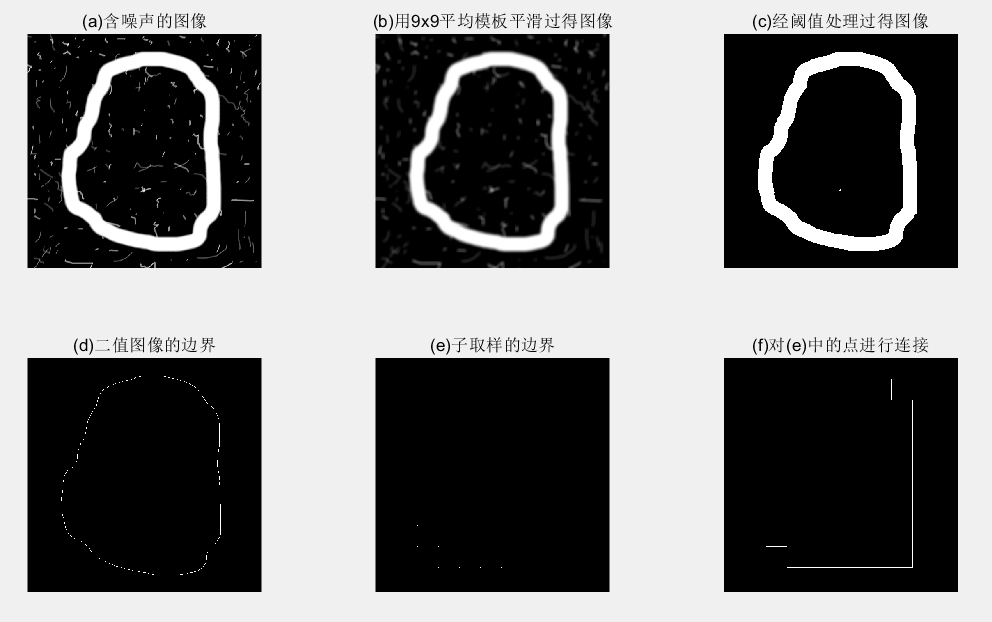1. 图(a)显示了一幅 570x570 大小的在镜面反射噪声中嵌入的圆形斧子图像f，这个例子的目的是获得物体外边界的链码和一阶差分。观察图 (a)，很明显，依附在物体上的噪声将导致一条很不规则的边界，它不是物体一般形状的真实描述。 当对噪声边界进行处理时，平滑通常是最常见的处理方式。
2. 图 (b)显示了使用 9x9平均模板的处理结果 ，图 ©所示的二值图像是经阈值处理后获得的，使用大约等于图像宽度 10%的网格进行分离。结果点可以显示为一幅图像，如图(e)所示。或使之成为连接序列(见图(f)),与图 (d)相反，通过比较两幅图，为链码采用这种表示方案的优点是很明显的。链码可以从标定过的序列 su 获得。

#### 2.2 使用最小周长多边形的多边形近似

1. 点合成法
2. 边分裂法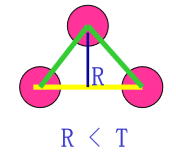1. 沿着边界选两个相邻的点对，计算首尾连接直 线段与原始折线段的误差R。
2. 如果误差R小于预先设置的阈值T。去掉中间 点，选新点对与下一相邻点对，重复1；否 则，存储线段的参数，置误差为0，选被存储线 段的终点为起点，重复1 2。
3. 当程序的第一个起点被遇到，算法结束。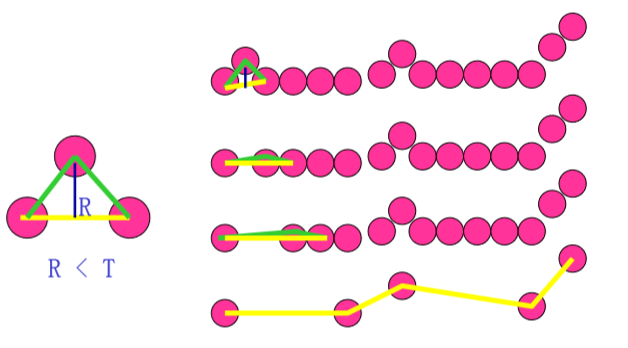1. 顶点一般不对应于边界的拐点（如拐角）。因为新的线段直到超过误差的阈值才开始画.
2. 例如：如果沿着一条长的直线追踪，而它出现了一个拐角，在超过阈值之前，拐角上的一些点会被丢弃 。分裂法可以缓解这个问题。

1. 连接边界线段的两个端点（如果是封闭边界，连接远点）；
2. 如果大正交距离大于阈值，将边界分为 两段，大值点定位一个顶点。重复1；
3. 如果没有超过阈值的正交距离，结束。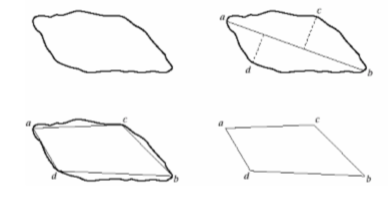MPP算法：

1. 表示边界有明显优势；
2. 可以进行边界平滑；

MPP算法实现总用到的一些M函数:

Q = qtdecomp(B, threshold, [mindim maxdim])

Q 是一个包含四叉树结构的稀疏矩阵，若Q (k, m)非零，则(k, m)为分解中的坐上块，块的大小为Q(k, m)。

[vals, r, c] = qtgetblk(B, Q, mindim)

1. vals 是一个在B 的四叉树分解中包含 mindim xmindim 个块的值的数组
2. Q 是由函数qtdecomp返回的稀疏矩阵
3. 参数r 和c 是包含左上角的行和列坐标的向量

IN = inpolygon(X, Y, xv, yv)

1. X 和 Y 是包含待测点的x 和y 坐标的向量
2. xv 和yv是包含按顺时针和逆时针顺序安排的多边形顶点的x 和y 坐标的向量

[X, Y, R] = im2minperpoly(ff cellsize)

1. f是一幅包含单一区域或边界的二值输入图像
2. cellsize 是细胞联合体中用于形成边界的方形单元的大小。
3. 列向量 X 和 Y 包含 MPP 顶点的 x 和 y 坐标
4. 输出 R 是由细胞组 合体包围的区域的二值图像

#### 2.3 标记

[st, angle, x0, y0] = signature(b, x0, y0)

[THETA, RHO] = cart2pol(X, Y)

X 和 Y 是包含笛卡尔坐标点的坐标向量。向量 THETA 和 RHO 包含对应的极坐标中的角度和长度。

[THETA, RHO] = cart2pol(X, Y)

#### 2.4 边界片段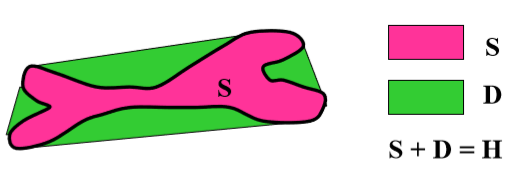#### 2.5 骨骼

Blum的中轴变换方法（MAT）：设:R是一个区域，B为R的边界点，对于R中 的点p，找p在B上“近”的邻居。如果p有多于一个的邻居，称它属于R的中轴（骨架）。

1. 不删除端点
2. 不破坏连通性
3. 不造成对区域的过度腐蚀

s = bwmorph(B, ‘skel’, Inf)

f = imread('D:\数字图像处理\第十一章学习\Fig1113(a).tif');
f = im2double(f);
h = fspecial ('gaussian',25,15);
g1 = imfilter(f, h, 'replicate');
g = im2bw(g1, 1.5*graythresh(g1));
s = bwmorph(g, 'skel', Inf) ;
s1 = bwmorph(s, 'spur', 8);
s2 = bwmorph(s1, 'spur', 7);
subplot(2,3,1);imshow(f);title('(a)分割后的人类染色体');
subplot(2,3,2);imshow(g1);title('(b) 使用 25 *25 高斯空间掩模平滑原图像的结果');
subplot(2,3,3);imshow(g);title('(c)经阈值处理后的图像');
subplot(2,3,4);imshow(s);title('(d)骨骼');
subplot(2,3,5);imshow(s1);title('(e)应用8次去除毛刺的算法后的图像');
subplot(2,3,6);imshow(s2);title('(f)再应用7次去除刺算法后的骨骼');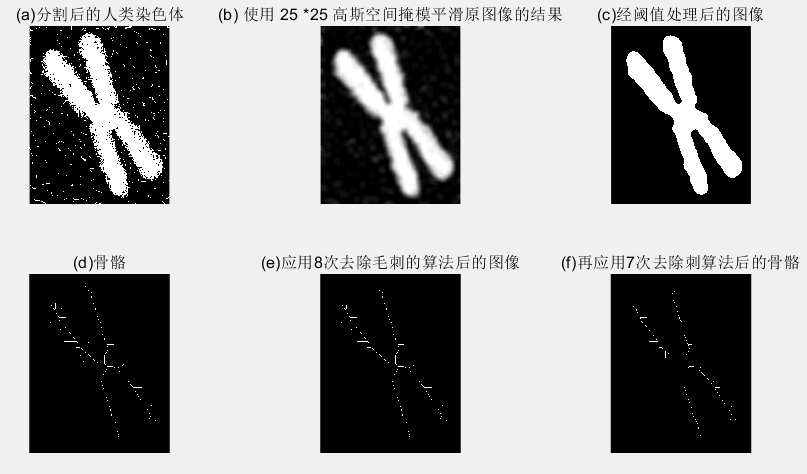1. 处理的第一步必须将染色体从与细节无关的背景中分离出来。一种方法是对图像进行平滑，然后进行阈值处理。图 (b)显示了对图像 f 使用 25x25、sig=5 的高斯空域模板进 行平滑后的结果。
2. 接下来，对平滑后的图像进行阈值处理，其中，自动确定阈值，将 graythresh (g) 乘以 1.5 以增加 50%的阈值处理总量。原因在 于，增加阈值将增加从边界中去除的数据数量，因而可以进一步减少噪声。
3. 重复上述操作 8 次。在这种情况下，近似等于 sig 的 1/2。一些小的刺状突起仍然存在于骨骼中。然而，再运用以上函数(以完成 sig 的值)7 次，产生的结果如图 (f) 所示，此结果是输入的合理骨骼表示。作为经验法则，高斯平滑模板的 sig 值对刺状突起去除算法的次数选择是不错的指导。

## （三）边界描述子

#### 3.1 一些简单的描述子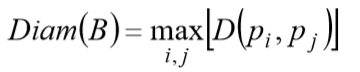D是欧氏距离或几何距离，pi, pj是边界上的 点。直径的长度和直径的两个端点连线（这条线被称为边界的主轴）的方向，是关于边界的有用的描 述符。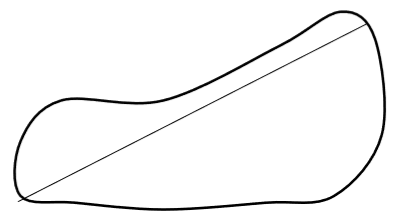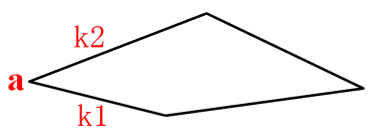k1、k2 为相邻线段的斜率

#### 3.2 形态数

循环首差为：33133030
循环首差：1 -2 = -1(3)      3 -0 = 3
0 -1 = -1(3)     3 -3 = 0
1 -0 = 1           2 -3 = -1(3)
0 -1 = -1(3)     2 -2 = 0

1. 序号4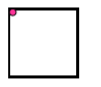链码：0321
首差：3333
形状：3333
2. 序号6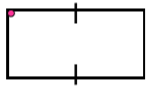链码：003221
首差：303303
形状：033033
3. 序号8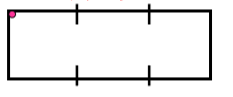链码：00032221
首差：30033003
形状：00330033

1. 首先确定形状数的序号n；

2. 在序号为n的矩形形状数中，找出一个与 给定形状的基本矩形的离心率接近的形状数。

3. 然后再用这个矩形与基本矩形对齐， 构造网格。

4. 用获得链码的方法得到链码。

5. 再得到循环首差。

6. 首差中的小循环数即为形状数。

#### 3.3 傅立叶描述子

1. 对于XY平面上的每个边界点，将其坐标用复数 表示为：s(k) = x(k) + jy(k)，k=0,1,…,N-1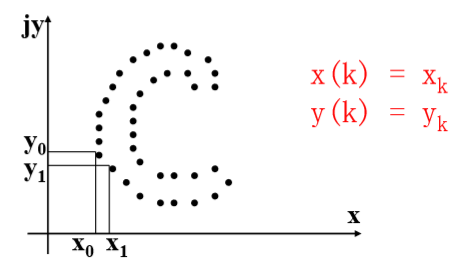2. 进行离散傅里叶变换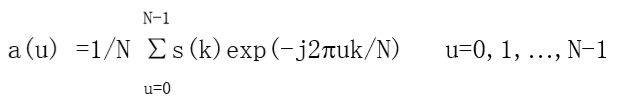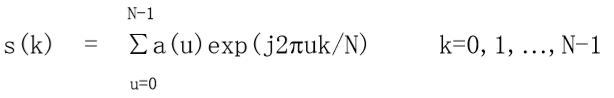系数a(u)被称为边界的傅里叶描述子。
3. 选取整数P小于等于N-1，进行逆傅里叶变换 （重构）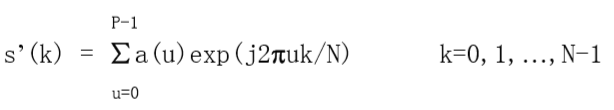这时，对应于边界的点数没有改变，但在重构每一个点所需要的计算项大大减少了。如果边界点数很大，P一般选为2的指数次方的整数。

P的选取与描述符的关系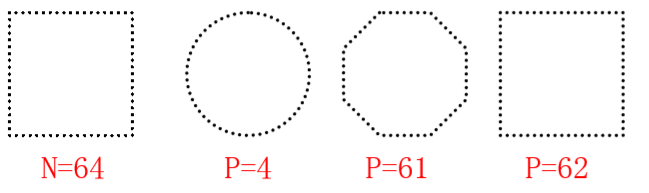1. 较少的傅里叶描述子（如4个），就可以获取边界本质的整体轮廓 。
2. 这些带有边界信息的描述子，可以用来区分明显不同的边界。

3. 使用复数作为描述符，对于旋转、平移、缩放等操作和起始点的选取不十分敏感。
4. 几何变换的描述子可通过对函数作简单变换来获得: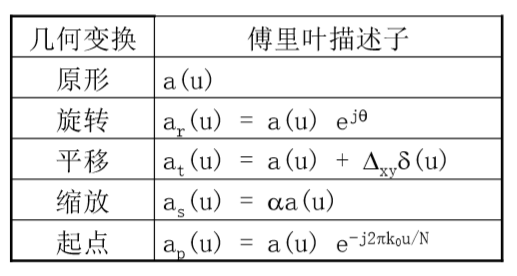#### 3.4 统计矩

1. 低阶矩描述图像的整体特征
2. 零阶矩反应目标的面积
3. 一阶矩反应目标的质心位置
4. 二阶矩反应了目标的主轴、辅轴的长短和主轴的方向角。
5. 高阶矩主要描述了图像的细节，如目标的扭曲度和峰态的分布等。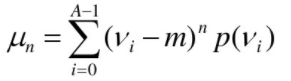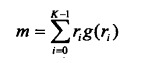m为Vi平均值，0矩阵为1，一矩阵为0，二阶矩度量曲线在均值附近的扩展程度，三阶矩度量曲线在均值附近的对称性。

## （四）区域描述子

#### 4.1 函数regionprops

D=regionprops(L,properties)

L是一个标记矩阵，D是长度max(L( : ))的一个结构

#### 4.2 纹理

1. 统计方法考察纹理的平滑、粗糙、粒状等特征；
2. 结构方法考察纹理的排列描述；处理图像像元的排列；
3. 频谱方法通过识别频谱中高能量的窄波峰寻找图像中的整体周期性。基于傅里叶频谱的特性。

#### 4.3 不变矩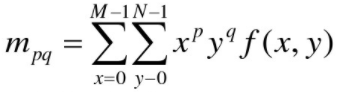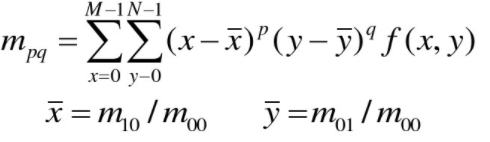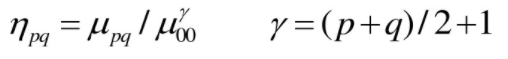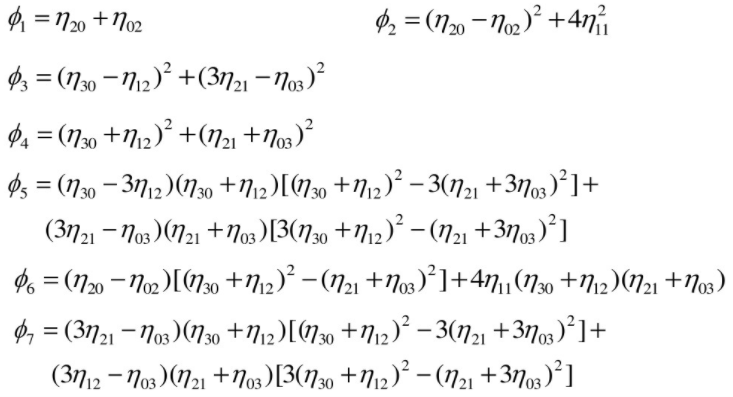## （四）主分量描述

f1 = imread('D:\数字图像处理\第十一章学习\Fig1130(a).tif');
subplot(2,3,1);imshow(f1,[ ]);title('(a可见蓝光波段');
subplot(2,3,2);imshow(f2,[ ]);title('(b)可见绿光波段');
subplot(2,3,3);imshow(f3,[ ]);title('(c)可见红光波段');
subplot(2,3,4);imshow(f4,[ ]);title('(d)近红外波段');
subplot(2,3,5);imshow(f5,[ ]);title('(e)中红外波段');
subplot(2,3,6);imshow(f6,[ ]);title('(f)热红外波段');

f1 = imread('D:\数字图像处理\第十一章学习\Fig1130(a).tif');
s=cat(3,f1,f2,f3,f4,f5,f6);
[ X , R ] = imstack2vectors(s);
P = principalcomps(X, 6);
gl = P.Y(:, 1);
gl = reshape(gl, 512, 512);
g2 = P.Y(:, 2);
g2 = reshape(g2, 512, 512);
g3 = P.Y(:, 3);
g3 = reshape(g3, 512, 512);
g4 = P.Y(:, 4);
g4 = reshape(g4, 512, 512);
g5 = P.Y(:, 5);
g5 = reshape(g5, 512, 512);
g6 = P.Y(:, 6);
g6 = reshape(g6, 512, 512);
subplot(2,3,1);imshow(gl,[ ]);title('(a可见蓝光波段主分量图像');
subplot(2,3,2);imshow(g2,[ ]);title('(b)可见绿光波段主分量图像');
subplot(2,3,3);imshow(g3,[ ]);title('(c)可见红光波段主分量图像');
subplot(2,3,4);imshow(g4,[ ]);title('(d)近红外波段主分量图像');
subplot(2,3,5);imshow(g5,[ ]);title('(e)中红外波段主分量图像');
subplot(2,3,6);imshow(g6,[ ]);title('(f)热红外波段主分量图像');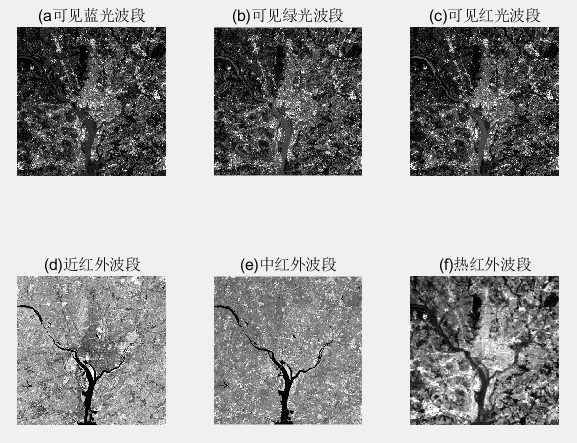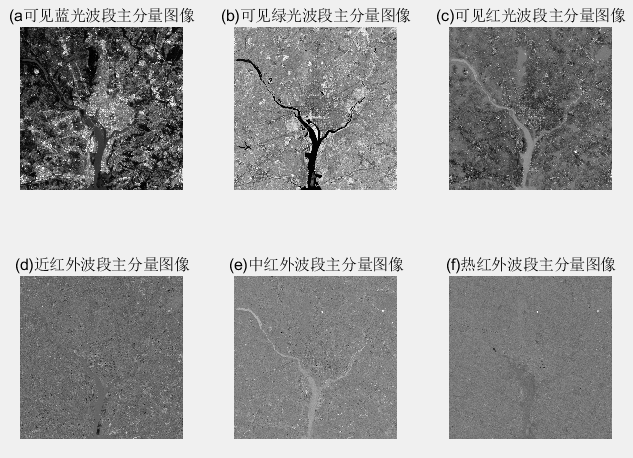f = imread('D:\数字图像处理\第十一章学习\Fig1134(a).tif');
[xl x2] = find(f);
X = [xl x2];
P = principalcomps(X, 2);
A = P.A;
Y = (A*(X'))';
minyl = min(Y(:, 1));
miny2 = min(Y(:, 2));
yl = round(Y(:, 1) - minyl + min(xl));
y2 = round(Y(:, 2) - miny2 + min(x2));
idx = sub2ind(size(f),yl,y2);
fout = false(size(f));
fout(idx) = 1;
fout = imclose(fout, ones(3));
fout = rot90(fout, 2);
subplot(1,2,1);imshow(f);title('(a)原始字符');
subplot(1,2,2);imshow(fout);title('(b)用主分量排列的字符');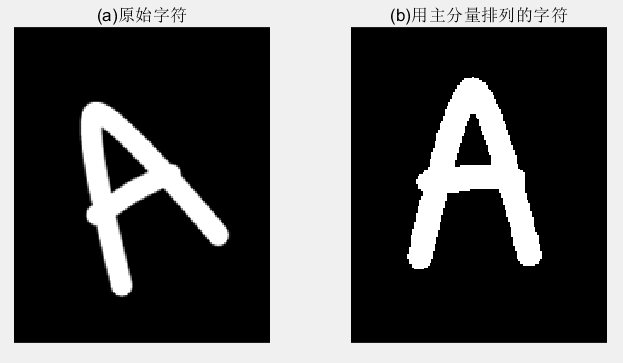©️2019 CSDN 皮肤主题: 技术工厂 设计师: CSDN官方博客